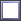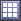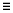# Review Evaluation Results

Review the input variable and output response values for each run, as well as review the run files.

## View Run Data Summary

View a detailed summary of all input variable and output response run data in a tabular format from the Evaluation Data tab.

1. From the Evaluate step, click the Evaluation Data tab.
2. From the Channel selector, select the channels to display in the summary table.
3. Analyze the run data summary.
4. Optional: Disable run data from post processing without deleting it entirely from the study by clearing a run's corresponding checkbox in the Post Process column.
When a run is disabled, it will be removed from all plots, tables, and calculations in the Post Processing step.

## Analyze Evaluation Plot

Plot a 2D chart of the input variable and output response values for each run using the Evaluation Plot tool.

1. From the Evaluate step, click the Evaluation Plot tab.
2. From the Channel selector, select the input variable and/or output response to plot along the y-axis.
The x-axis represents the run numbers.
3. Analyze the plot.

## Analyze Dependency Between Two Sets of Data

Analyze the dependency between two sets of data in a scatter plot from the Evaluation Scatter tab. Visually emphasize data in the scatter plot by appending additional dimensions in the form of bubbles.

1. From the Evaluate Step, click the Evaluation Scatter tab.
2. Select data to display in the scatter plot.
• Use the Channel selector to select two dimensions of data to plot.
• Use the Bubbles selector to select additional dimensions of data to visually emphasize in the scatter plot. The selected input variables/output responses are represented by varying sizes and colors of bubbles.
The size and color of bubbles is determined by values in the run data for the selected input variable/output response. For size, larger bubbles equal larger values. For color, different shades of red, blue, and gray are used to visualize the range of values. The darker the shade of red, the larger the value. The lighter the shade of blue, the smaller the value. Gray represents the median value.
3. Analyze the dependencies between the selected data sets.
Tip: Display selected data in a single plot or separate plots by switching the Multiplot option between(single plot) and(multiple plots).
Configure the scatter plot's display settings by clicking(located in the top, right corner of the work area). For more information about these settings, refer to Evaluation Scatter Tab Settings.

## View Iteration History Summary

View a detailed iteration history summary of all input variables and output responses in a tabular format using the Iteration History tool.

1. From the Evaluate step, click the Iteration History tab.
2. From the Channel selector, select the channels to display in the table.
3. Analyze the iteration history summary.

### Iteration History Table Data

Data reported in the Iteration History table.

#### General Column Data

Iteration Index
Displays the current iteration number.
Evaluation Reference
Corresponds to the row number in the Evaluation Data table.
Iteration Reference
Displays the iteration number where the current optimal is located.
Condition
Identifies one of three states for the iterate:
• Violated. At least one constraint is violated.
• Feasible. No constraints are violated.
• Acceptable. At least one constraints violated, but only by a very small percentage.
Best Iteration
Identifies the set of iterations on the non-dominated Pareto front; this is the optimal set of solutions in a multi-objective optimization.
Note:
• Only applicable to multi-objective problems.
• This column is populated once the evaluation is complete

#### System Identification Column Data

Additional columns are added to the Iteration History table if the objective of your study is system identification.
Objective Function Value
Sum of normalized difference-squared between the objective function value and target value.
DTV
Delta between the target value and the objective value for each objective.
DTVN
Normalized DTV for each objective.

#### Probabilistic Method Column Data

When using a probabilistic method, additional columns are added to the Iteration History table. The probabilistic method channels are defined in Table 1.

Table 1.
Channel SORA and SORA_ARSM SRO
Objective label The value of the objective at the given input variable values. The value of the objective at the given input variable values.
Objective label (value at percentile) The estimated objective value at the specified robust min/max percent CDF. -
Standard Deviation of Objective - The measurement of the spread in the objective distribution.
Constraint label The value of the constraint at the given input variable values. The value of the constraint at the given input variable values.
Constraint label (value at percentile) The estimated constraint value at the constrained CDF limit. The reliability of design with respect to this constraint.
System reliability - The reliability of the system considering all constraints.

The value of the constraint that meets the required reliability.

Consider a case where a constraint value needs to be less than 75.0 with 98% reliability. In the first iteration, Sequential Optimization and Reliability Assessment finds a design with a constraint value of 75.025, but the PV value for 98% reliability is at 99.383. Hence, this design meets the constraint's upper bound, but does not meet the reliability requirement. In the sixth iteration, Sequential Optimization and Reliability Assessment finds a design with a constraint value of 57.412 and the PV value for 98% reliability is at 75.075. This design meets the reliability constraint, as 98% of the design will have a constraint value less than 75.075.

#### Column Color Coding

The Iteration History table uses color coding to help you determine which designs are feasible, optimal, and violated.
Note: If an iteration contains a violated constraint, the violated constraint is displayed in a bold font.
White Background/Black Font
Feasible design
White Background/Red Font
Violated design
White Background/Orange Font
Acceptable design, but at least one constraint is near violated
Green Background/White Font
Optimal design
Green Background/Orange Font
Optimal design, but at least one constraint is near violated

## Analyze Iteration Plot

Plot the iteration history of a study's objectives, constraints, input variables and unused output responses in a 2D chart using the Iteration Plot tab.

1. From the Evaluate step, click the Iteration Plot tab.
2. From the Channel selector, select the input variable and/or output response to plot along the y-axis.
The x-axis represents the iteration history.
3. Analyze the plot.
When the objective history is plotted, infeasible designs are marked with larger markers. When the constraint history is plotted, the constraint bounds can be marked with a datum line.
Tip: To display datum lines, click(located in the top, right corner of the work area) and enable Bounds.